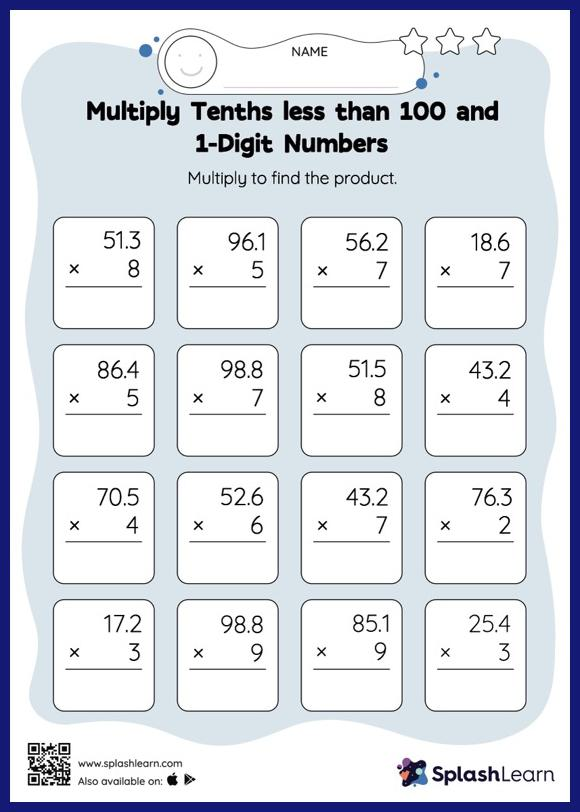# Multiply Tenths less than 100 and 1-Digit Numbers: Vertical Multiplication Worksheet

Home > Multiply Tenths less than 100 and 1-Digit Numbers: Vertical MultiplicationThis multiply tenths less than 100 and 1-digit numbers worksheet has a set of problems curated to ensure that your child becomes more proficient in multiplication. When multiplying a decimal by a one-digit number, students may ignore the decimal point during calculations and bring it back when writing the result. This multiply tenths less than 100 and 1-digit numbers worksheet provides plenty of practice with this concept. In this worksheet, numbers are laid one on top of another (vertical format). This encourages students to use the inherent place value structure to solve the problems.